Macros

Accounts Tax
Excel

To convert Amount into Words in Indian Currency, we have make a formula.

To make a formula, we use Excel Macros.

Follow the steps given below to use the formula

## Steps to make Excel Formula

1. Open excel file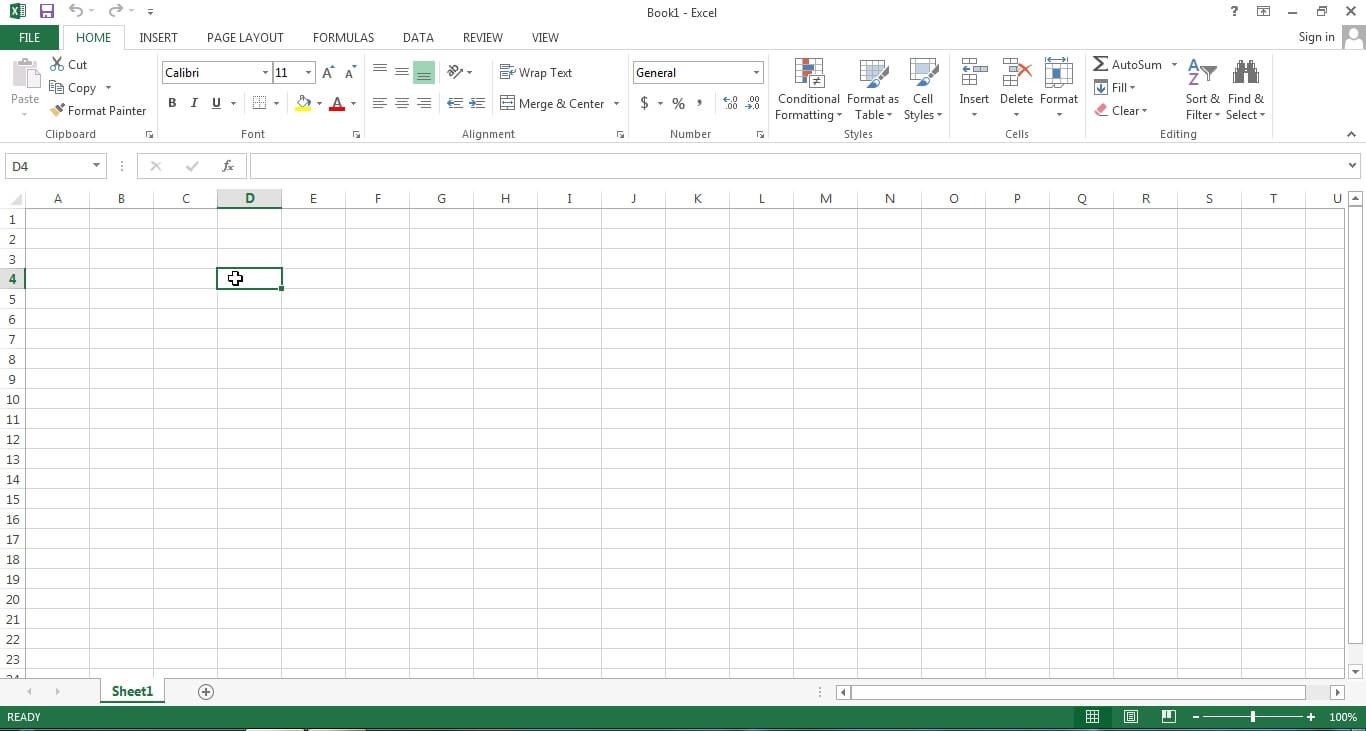2. Press Alt F11 (It shows like)3. Insert --> Module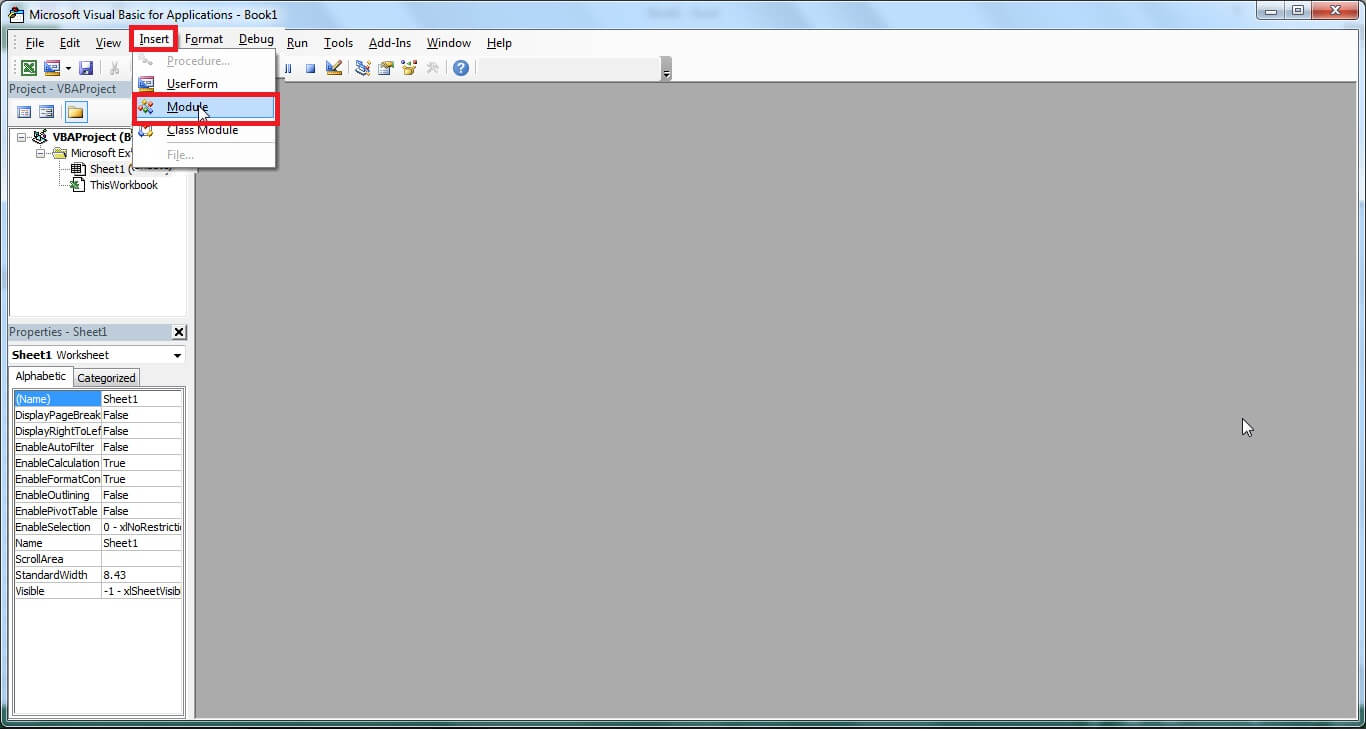4. Copy paste the following code from here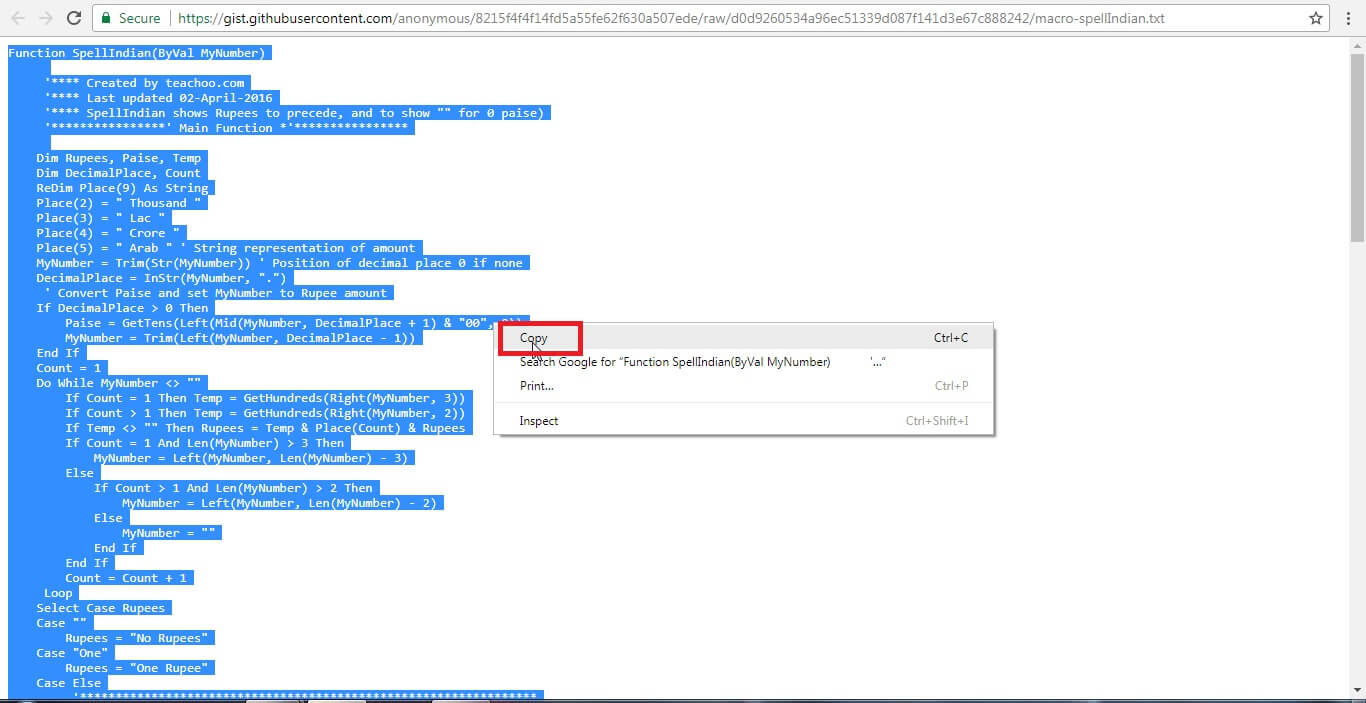Function SpellIndian(ByVal MyNumber)

'**** Created by teachoo.com
'**** Last updated 02-April-2016
'**** SpellIndian shows Rupees to precede, and to show "" for 0 paise)
'****************' Main Function *'****************

Dim Rupees, Paise, Temp
Dim DecimalPlace, Count
ReDim Place(9) As String
Place(2) = " Thousand "
Place(3) = " Lac "
Place(4) = " Crore "
Place(5) = " Arab " ' String representation of amount
MyNumber = Trim(Str(MyNumber)) ' Position of decimal place 0 if none
DecimalPlace = InStr(MyNumber, ".")
' Convert Paise and set MyNumber to Rupee amount
If DecimalPlace > 0 Then
Paise = GetTens(Left(Mid(MyNumber, DecimalPlace + 1) & "00", 2))
MyNumber = Trim(Left(MyNumber, DecimalPlace - 1))
End If
Count = 1
Do While MyNumber <> ""
If Count = 1 Then Temp = GetHundreds(Right(MyNumber, 3))
If Count > 1 Then Temp = GetHundreds(Right(MyNumber, 2))
If Temp <> "" Then Rupees = Temp & Place(Count) & Rupees
If Count = 1 And Len(MyNumber) > 3 Then
MyNumber = Left(MyNumber, Len(MyNumber) - 3)
Else
If Count > 1 And Len(MyNumber) > 2 Then
MyNumber = Left(MyNumber, Len(MyNumber) - 2)
Else
MyNumber = ""
End If
End If
Count = Count + 1
Loop
Select Case Rupees
Case ""
Rupees = "No Rupees"
Case "One"
Rupees = "One Rupee"
Case Else
'****************************************************************
'modified the following two lines to display "Rupees" to precede
' rem'd the first line and added the second line
'****************************************************************
'Rupees = Rupees & " Rupees"
Rupees = "Rupees " & Rupees

End Select
Select Case Paise
Case ""
'****************************************************************
'modified the following two lines to display nothing for no paise
' rem'd the first line and added the second line
'****************************************************************

'Paise = " and No Paise"
'****************************************************************
'modified the following line to display " Only" for no paise
' rem'd the first line and added the second line
'****************************************************************
'Paise = ""
Paise = " Only"
Case "One"
Paise = " and One Paisa"
Case Else
Paise = " and " & Paise & " Paise"

End Select
SpellIndian = Rupees & Paise
End Function
'*******************************************
' Converts a number from 100-999 into text *
'*******************************************
Function GetHundreds(ByVal MyNumber)
Dim Result As String
If Val(MyNumber) = 0 Then Exit Function
MyNumber = Right("000" & MyNumber, 3) 'Convert the hundreds place
If Mid(MyNumber, 1, 1) <> "0" Then
Result = GetDigit(Mid(MyNumber, 1, 1)) & " Hundred "
End If
'Convert the tens and ones place
If Mid(MyNumber, 2, 1) <> "0" Then
Result = Result & GetTens(Mid(MyNumber, 2))
Else
Result = Result & GetDigit(Mid(MyNumber, 3))
End If
GetHundreds = Result
End Function
'*********************************************
' Converts a number from 10 to 99 into text. *
'*********************************************
Function GetTens(TensText)
Dim Result As String
Result = "" ' null out the temporary function value

If Val(Left(TensText, 1)) = 1 Then ' If value between 10-19
Select Case Val(TensText)
Case 10: Result = "Ten"
Case 11: Result = "Eleven"
Case 12: Result = "Twelve"
Case 13: Result = "Thirteen"
Case 14: Result = "Fourteen"
Case 15: Result = "Fifteen"
Case 16: Result = "Sixteen"
Case 17: Result = "Seventeen"
Case 18: Result = "Eighteen"
Case 19: Result = "Nineteen"
Case Else
End Select
Else ' If value between 20-99
Select Case Val(Left(TensText, 1))
Case 2: Result = "Twenty "
Case 3: Result = "Thirty "
Case 4: Result = "Forty "
Case 5: Result = "Fifty "
Case 6: Result = "Sixty "
Case 7: Result = "Seventy "
Case 8: Result = "Eighty "
Case 9: Result = "Ninety "
Case Else
End Select
Result = Result & GetDigit _
(Right(TensText, 1)) 'Retrieve ones place
End If
GetTens = Result
End Function
'*******************************************
' Converts a number from 1 to 9 into text. *
'*******************************************
Function GetDigit(Digit)
Select Case Val(Digit)
Case 1: GetDigit = "One"
Case 2: GetDigit = "Two"
Case 3: GetDigit = "Three"
Case 4: GetDigit = "Four"
Case 5: GetDigit = "Five"
Case 6: GetDigit = "Six"
Case 7: GetDigit = "Seven"
Case 8: GetDigit = "Eight"
Case 9: GetDigit = "Nine"
Case Else: GetDigit = ""
End Select
End Function

5. Paste all text in module and select Spellindian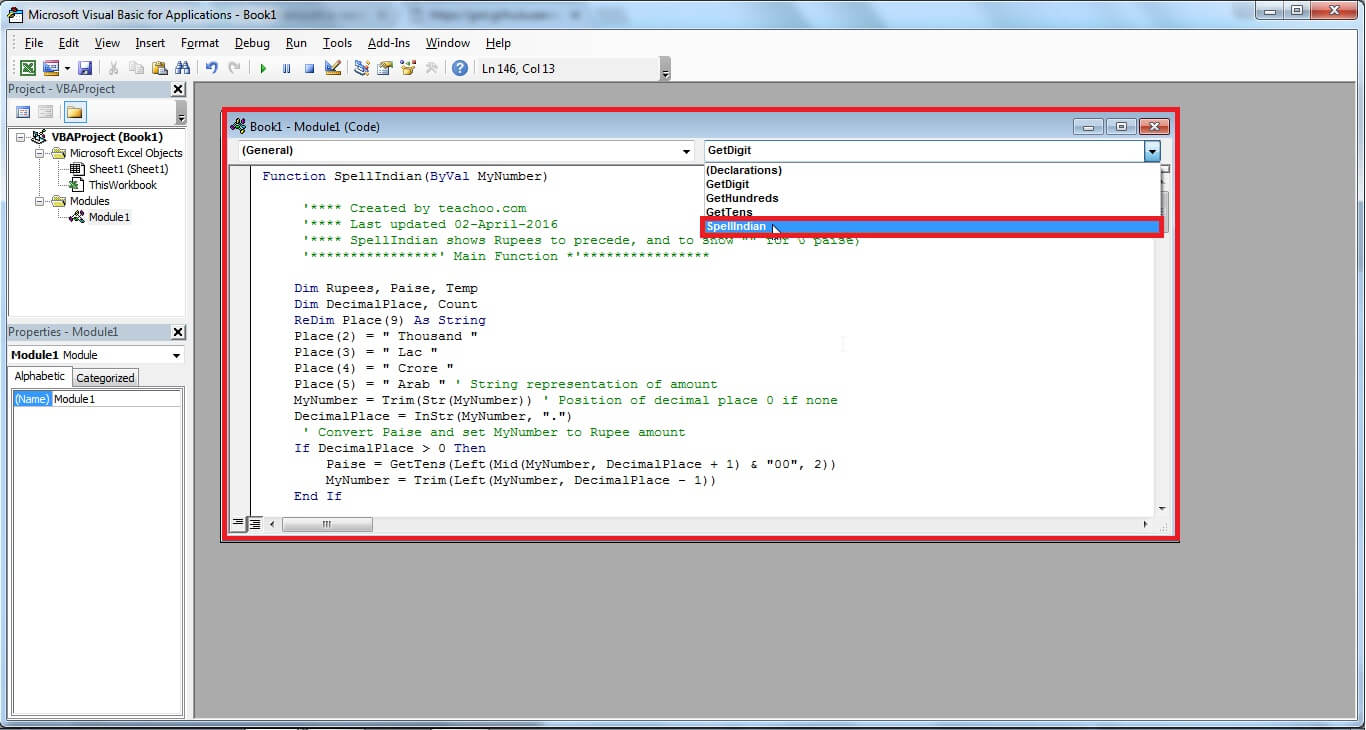6. SpellIndian (number) is the function which you can use in the excel file.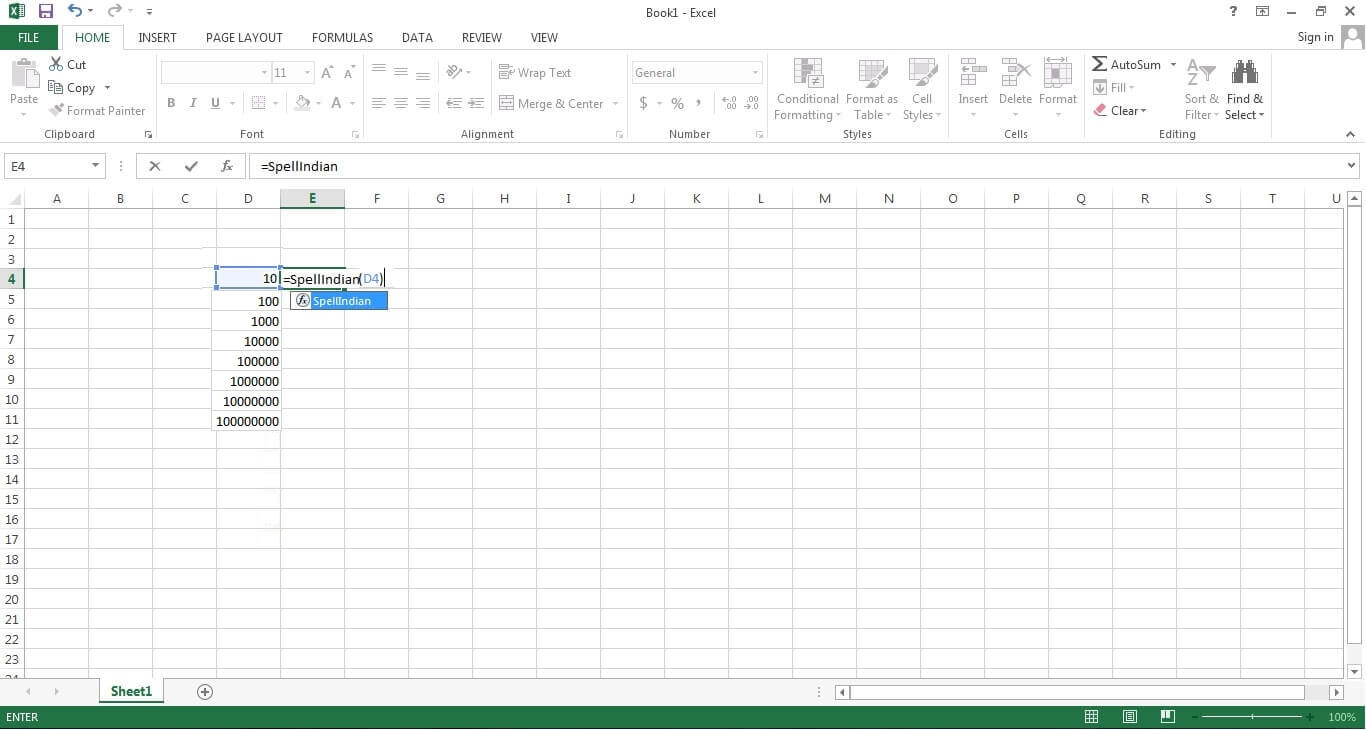7. It shows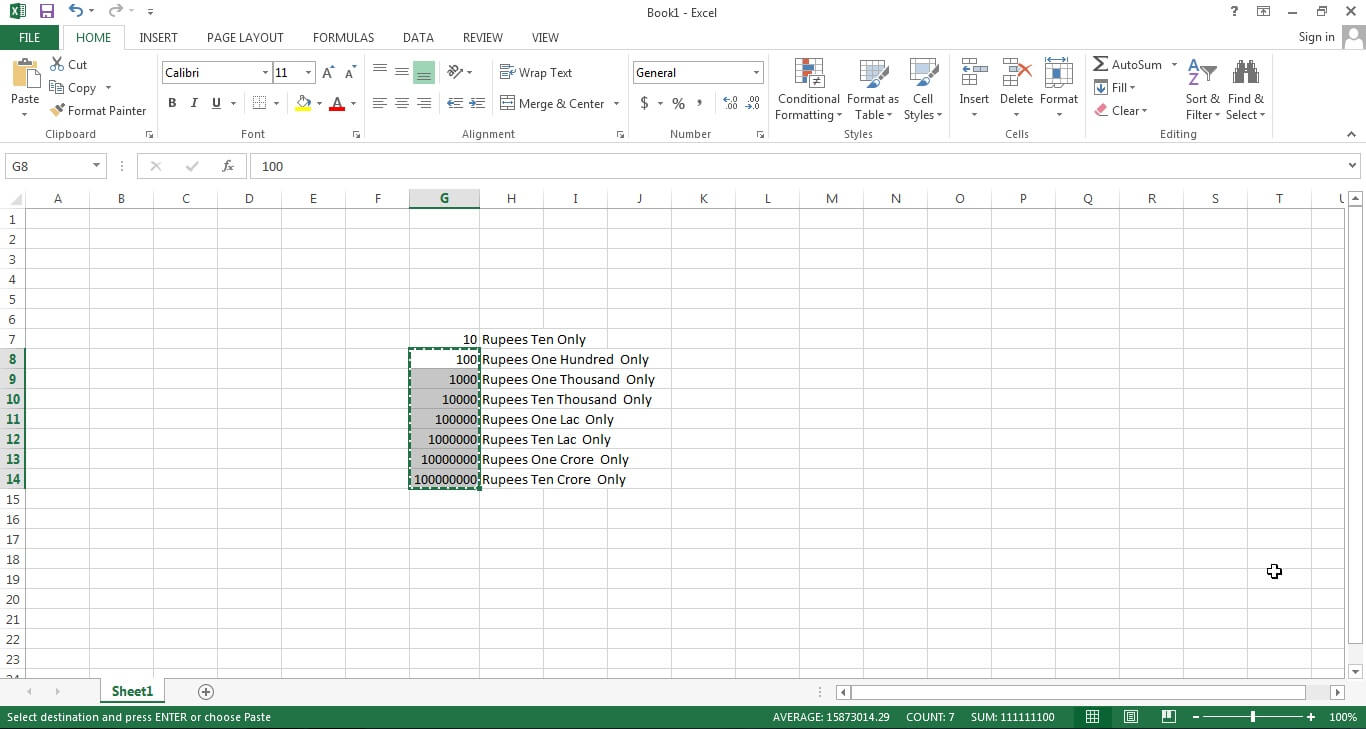Get live Maths 1-on-1 Classs - Class 6 to 12